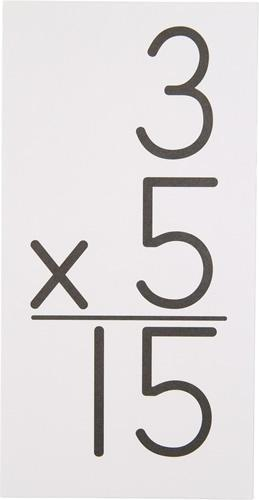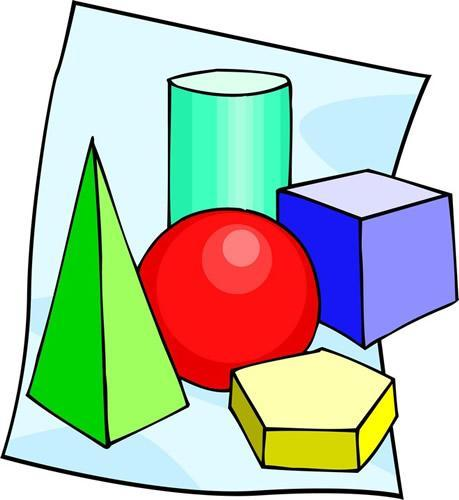# Mathematics & Computer Science

Evan Levy
Supervisor K-12

The purpose of the district mathematics program is to provide young students with experiences that will allow them to develop mathematical reasoning and acquire basic mathematical concepts and skills. The primary curriculum makes widespread use of manipulatives (concrete objects) for deeper understanding.
The curriculum is designed to provide students with growth in competencies essential to mathematical growth. As they learn mathematics, they will develop increasingly sophisticated problem-solving skills, a range of mathematical “habits of mind,” and increasing sophistication in mathematical reasoning. In addition, students will become increasingly proficient in oral and written mathematical expression, as they gain fluency in the language of mathematics and ability to make connections within mathematics.

Elementary (Grades 3 - 5)The mathematics program in the intermediate grades focuses on the district’s goals of understanding mathematical concepts and the development of critical thinking skills. As the students progress through the grades, the skills they have learned in grades K–2 are reinforced and new concepts are introduced.
Students learn mathematics through a problem solving, inquiry, and discovery based approach. They use communication to organize and clarify their mathematical thinking and recognize that mathematical facts, procedures, and claims must be justified. Students create and use representations to organize, record, and communicate mathematical ideas.

Middle School (Grades 6 - 8)